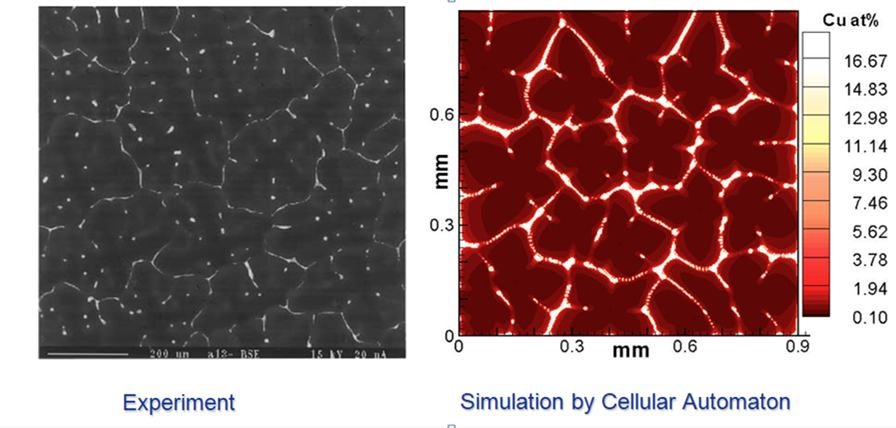## PanEngine API

PanEngine API view documentations

PanEngine API is a dynamic-linked library that can be integrated with user’s in-house code to create custom applications such as solidification, heat-treatment, casting, welding, corrosion and phase field simulation. PanEngine is currently built as a Dynamic Link Library (DLL) in Windows, which is a collection of functions for calculating thermodynamic, thermo-physical properties and phase equilibria for a multi-component, multi-phase system. It implements a set of numerical algorithms for solving nonlinear equations and unconstrained/constrained optimization problems involved in multi-phase phase equilibrium for a multi-component system. It should be emphasized that the specially designed global minimization algorithm enables PanEngine to find the most stable phase equilibrium in a more intelligent and reliable way. Moreover, it finds the correct, stable phase equilibria without requiring the user to input initial values. This feature is critically important especially when the thermodynamic calculation engine is integrated with processing simulation software since it is impossible for the user to provide initial values and verify the results for thousands of points during the simulation. On the other hand, for multi-component systems, it is almost impossible for the user to provide proper initial values for stable phase equilibria.

Basic Functionalities of PanEngine API

• Load database and select components: loads databases in tdb or pdb format
• Calculate global/local equilibrium: automatic calculates global equilibrium or local equilibrium as required
• Find liquidus surface: finds liquidus temperature for a given composition
• Calculate liquidus slope: calculates liquidus slope along specified direction
• Calculate partition coefficient: calculates partition coefficient for a component between two phases in equilibrium
• Calculate thermodynamic factor: calculates thermodynamic factors for selected components
• Solidification simulation: performs solidification simulation with selected model

Featured Plots of PanEngine APIThis figure shows an example PanEngine application which couples with Cellular Automaton for the simulation of microstructure evolution of Al-3.9Cu-0.9Mg (wt%) alloy solidified at 0.78 K/s. The left figure is experimentally observed microstructure.